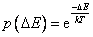Home News About Me Ph. D. Thesis Abstract Table of Contents 1. Introduction 2. Theory  Fundamentals of the Multivariate Data Analysis 2.1. Overview of the Multivariate Quantitative Data Analysis 2.2. Experimental Design 2.3. Data Preprocessing 2.4. Data Splitting and Validation 2.5. Calibration of Linear Relationships 2.6. Calibration of Nonlinear Relationships 2.7. Neural Networks  Universal Calibration Tools 2.8. Too Much Information Deteriorates Calibration 2.8.1. Overfitting, Underfitting and Model Complexity 2.8.2. Neural Networks and the Complexity Problem 2.8.3. Brute Force Variable Selection 2.8.4. Variable Selection by Stepwise Algorithms 2.8.5. Variable Selection by Genetic Algorithms 2.8.6. Variable Selection by Simulated Annealing 2.8.7. Variable Compression by Principal Component Analysis 2.8.8. Topology Optimization by Pruning Algorithms 2.8.9. Topology Optimization by Genetic Algorithms 2.8.10. Topology Optimization by Growing Neural Network Algorithms 2.9. Measures of Error and Validation 3. Theory  Quantification of the Refrigerants R22 and R134a: Part I 4. Experiments, Setups and Data Sets 5. Results  Kinetic Measurements 6. Results  Multivariate Calibrations 7. Results  Genetic Algorithm Framework 8. Results  Growing Neural Network Framework 9. Results  All Data Sets 10. Results  Various Aspects of the Frameworks and Measurements 11. Summary and Outlook 12. References 13. Acknowledgements Publications Research Tutorials Downloads and Links Contact Search Site Map Print this Page### 2.8.6.   Variable Selection by Simulated Annealing

Simulated Annealing (SA) is a method that simulates the thermodynamic process in which metal is heated to its melting temperature and then slowly cooled to its crystal configuration of lowest energy. The system is in thermal equilibrium when the probability of a certain state is governed by a Boltzmann distribution:(18)

with E as energy, T as temperature and k as Boltzmann's constant. Kirkpatrick et al.  applied SA to an optimization problem. During the minimization of a multivariate function, a candidate solution is generated by randomly perturbing the current configuration and the energy (similar to the fitness of GA) is calculated. If the new energy is lower than the current, the displacement is accepted. If the energy is higher, the displacement is accepted with a probability given by the Boltzmann distribution . These uphill steps allow the algorithm to escape from local minima. The probability of accepting an uphill step is a function of the change of the energy and of the temperature, which is gradually lowered during the search process. Due to the similar approach with random steps in the search process, SA has several times been compared with GA for the selection of variables whereby SA achieved comparable or slightly worse results and consequently will not be used in this study -.

 Page 53 © Frank Dieterle, 03.03.2019